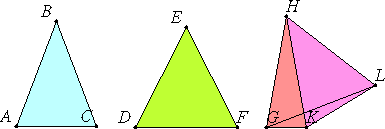# Proposition 22

If there are three plane angles such that the sum of any two is greater than the remaining one, and they are contained by equal straight lines, then it is possible to construct a triangle out of the straight lines joining the ends of the equal straight lines.

Let there be three plane angles ABC, DEF, and GHK, of which the sum of any two is greater than the remaining one, so that the sum of the angles ABC and DEF is greater than the angle GHK, the sum of the angles DEF and GHK is greater than the angle ABC, and, further, the sum of the angles GHK and ABC is greater than the angle DEF. Also let the straight lines AB, BC, DE, EF, GH, and HK be equal.

Join AC, DF, and GK.

I say that it is possible to construct a triangle out of straight lines equal to AC, DF, and GK, that is, that the sum of any two of the straight lines AC, DF, and GK is greater than the remaining one.Now, if the angles ABC, DEF, and GHK equal one another, then it is clear that, AC, DF, and GK also being equal, it is possible to construct a triangle out of straight lines equal to AC, DF, and GK.

But, if not, let them be unequal. Construct the angle KHL equal to the angle ABC at the point H on the straight line HK. Make HL equal to any one of the straight lines AB, BC, DE, EF, GH, or HK. Join KL and GL.

I.4

Now, since the two sides AB and BC equal the two sides KH and HL, and the angle at B equals the angle KHL, therefore the base AC equals the base KL.

And, since the sum of the angles ABC and GHK is greater than the angle DEF, while the angle ABC equals the angle KHL, therefore the angle GHL is greater than the angle DEF.

I.24

And, since the two sides GH and HL equal the two sides DE and EF, and the angle GHL is greater than the angle DEF, therefore the base GL is greater than the base DF.

But the sum of GK and KL is greater than GL. Therefore the sum of GK and KL is much greater than DF.

But KL equals AC, therefore the sum of AC and GK is greater than the remaining straight line DF.

Similarly we can prove that the sum of AC and DF is greater than GK, and further, the sum of DF and GK is greater than AC. (I.22)

Therefore it is possible to construct a triangle out of straight lines equal to AC, DF, and GK.

Therefore, if there are three plane angles such that the sum of any two is greater than the remaining one, and they are contained by equal straight lines, then it is possible to construct a triangle out of the straight lines joining the ends of the equal straight lines.

Q.E.D.

## Guide

This construction is the first stage of the construction in the next proposition to make a solid angle given three plane angles.

The proof succeeds in showing that if each of the three plane angles is less than the sum of the other two, then each of the three lines AC, DF, and DK is less than the sum of the other two. The latter is a necessary condition for a triangle to be made with its three sides equal to those three lines according to I.20. But it was never shown to be sufficient to make such a triangle in I.22, and it is that sufficiency which is being invoked in this proof. Thus, there is a serious flaw in the proof.